Next: Josephson Junction Model Up: Superconductor Devices Previous: Superconductor Devices   Contents   Index

## Josephson Junctions

General Form:
bname n+ n- [np] [modname] [parameters ...]

Parameter Name Description
pijj=1|0 Whether the device is a pi'' junction.
area=val Scale factor that multiplies all currents and other values, effectively modifying the junction area.
ics=val Instantiated critical current, used as scale factor for capacitance, conductances.
lser=val Junction series parasitic inductance.
lsh=val Shunt resistor series parasitic inductance.
ic=vj,phi The initial junction voltage and phase (initial condition) for transient analysis.
vj=vj The initial junction voltage (initial condition) for transient analysis, alias ic_v.
phi=phi The initial junction phase (initial condition) for transient analysis, alias ic_phase.
control=name Controlling voltage source or inductor name.

Examples:
b1 1 0 10 jj1 ics=200uA
bxx 2 0 type1 control=l3
b2 4 5 ybco phi=1.57

Unless stated otherwise, information presented here also applies to The Verilog-A Josephson junction model provided with WRspice in the Verilog-A examples.

pijj If the pijj parameter is given and set to a nonzero integer value, the device instance will behave as a pi'' junction. This type of junction has a ground state with phase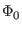rather than 0. The value given on the device line (if any) overrides the value given in the model. This parameter may not be recognized in the Verilog-A model.

area
range: 0.05 - 20.0
Deprecated, do not use in new files.
Histrorically, this parameter has been used to set the actual critical current of a Josephson junction instance. It is not a physical area, but rather a scale factor, representing the ratio of the instance critical current to the reference critical current. The parameter is retained for backwards compatibility, but should not be used in new circuit descrfiptions. The ics parameter (below) should be used instead. By using ics, one can change the critical current of the reference junction without changing the instance critical currents, which is not the case for area. In the new paradigm, the reference junction critical current corresponds to a typical'' mid-sized junction, with a not necessarily convenient critical current value. Use of area assumes that the reference critical current is something nice, like the historical 1mA, and unchanging. If not specified and ics is not given, the effective value is 1.0.

ics
range: 0.05*icrit - 20.0*icrit
This gives the actual critical current of the instantiated junction, and in addition scales all conductance and capacitance values from the reference junction appropriately. This is equivalent to giving the area parameter with a value of ics/icrit. The default is icrit, the reference junction critical current.

lser
range: 0.0 - 10.0pH
This models series inductance of the physical Josephson junction structure, caused by constriction of current through the junction orifice. This inductance might typically be in the range of 0.1 to 0.3 picohenries. If nonzero, an internal node is added to the model, providing the connection point of the inductance and the Josephson junction. The default value is 0.0, meaning that no parasitic inductance is assumed. Nonzero given values less than 0.01pH revert to zero.

lsh
range: 0.0 - 100.0pH
This parameter specifies the series inductance of the external shunt resistance. The vshunt model parameter must be specified such that a positive external shunt conductance is applied, otherwise this parameter is ignored. Ordinarily, the lsh0/lsh1 model parameters would be used to specify the inductance, this parameter can be used to override these values on a per-instance basis if desired.

ic
Presently, this keyword is not recognized by the Verilog-A model. The keyword is expected to be followed by two numbers, giving the initial junction voltage and phase in radians. These apply in transient analysis when the uic'' option is included in the transient analysis specification. The initial junction voltage and phase both default to 0.0.

vj or ic_v
This provides the initial voltage of the junction when the uic'' option is included in the transient analysis specification. The initial junction voltage defaults to 0.0.

phi or ic_phase
This provides the initial junction phase in radians when the uic'' option is included in the transient analysis specification.

control
This keyword is not recognized by the Verilog-A model. The control parameter is only needed if critical current modulation is part of the circuit operation, and is only relevant to Josephson junction model types that support critical current modulation, that is, the model parameter cct is given a value larger than 1. The name in the control specification is the name of either a voltage source or inductor which appears somewhere in the circuit. The current flowing through the indicated device is taken as the junction control current.

The Josephson junction device has unique behavior which complicates simulation with a SPICE-type simulator. Central is the idea of phase, which is a quantum-mechanical concept and is generally invisible in the non-quantum world. However with superconductivity, and with Josephson junctions in particular, phase becomes not only observable, but a critical parameter describing these devices and the circuits that contain them.

Without going into the detailed physics, one can accept that phase is an angle which applies to any superconductor. The angle is a fixed value anywhere on the superconductor, unless current is flowing. Flowing current produces magnetic flux, and magnetic flux produces a change in phase. One can express this as follows:

LI = flux =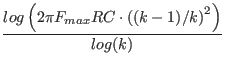(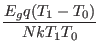/2)

Here, L is the inductance,is the magnetic flux quantum (Planck's constant divided by twice the electron charge) andis the phase difference across the inductor. The supercurrent flowing in a Josephson junction is given by

I = Icsin()

where Ic is the junction critical current, andis the phase difference across the junction. The junction phase is proportional to the time integral of junction voltage:= (2/)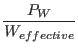V(t)dt

The important consequence is that the sum of the phase differences around any loop consisting of Josephson junctions and inductors must be a multiple of 2. This is due to the requirement that the superconducting wave function be continuous around the loop. Further, if the loop phase is not zero, it implies that a persistent current is flowing around the loop, and that the magnetic fluc through the loop is a multiple of the flux quantum.

We therefor observe that in a circuit containing loops of Josephson junctions and inductors, which includes about all useful circuits:

1. The DC voltage across each Junction or inductor is zero.
2. The DC current applied to the circuit splits in such a manner as to satisfy the phase relations above.

Without any built-in concept of phase, it would appear to be impossible to find the DC operating point of a circuit containing Josephson junctions and inductors with a SPICE simulator. However, there are ways to accomplish this.

The time-honored approach, used successfully for many years, is to skip DC analysis entirely. One generally is interested only in transient analysis, describing the time evolution of the circuit under stimulus, and a DC analysis would only be necessary to find the initial values of circuit voltages and currents. Instead of a DC analysis, what is done is every voltage and current source starts at zero voltage or current, and ramps to the final value in a few picoseconds. The transient analysis is performed using the use initial conditions'' (uic'') option, where there is no DC operating point analysis, and transient analysis starts immediately with any supplied initial condicions (which are not generally given in this case). By ramping up from zero, the phase condition around junction/inductor loops is satisfied via Kirchhoff's voltage law. Actually, this ensures that the loop phase is constant, but it is zero as we started from zero. Initially, there is no trapped flux'' in the inductor/junction loops, so assumption of zero phase is correct. Thus the prescription is to ramp up all sources from zero, use the uic option of transient analysis, and wait for any transients caused by the ramping sources to die away before starting the real'' simulation. The ramping-up effectively replaces the DC operating point analysis.

WRspice after release 4.3.3 offers a DC analysis capability which uses phase-mode for circuits containing Josephson junctions. Unlike strictly phase-mode simulators, WRspice allows a mixture of phase (inductors and Josephson junctions) and voltage mode components.

Within WRspice, every node connected to a Josephson junction, inductor, or lossless transmission line (treated as an inductor in DC analysis) has a Phase'' flag set. This indicates that the computed value is phase, not voltage, for these nodes. We have to special-case the matrix loading functions for inductors, mutual inductors, lossless transmission lines, and resistors. Other devices are treated normally.

A Josephson junction can be modeled by the basic formula

I = Icsin(V(i, j))

where V(i, j) is the voltage'' difference between nodes i and j (across the junction) which is actually the phase. Inductors look like resistors:

I =V(i, j)/2L

Where V(i, j) is the phase difference across the inductor. Mutual inductance adds similar cross terms.

Capacitors are completely ignored as in normal DC analysis. The treatment of resistors is slightly complicated. The connected nodes can be Ground'', Phase'', or Voltage'' type. If both nodes are Voltage or Ground, the resistor is loaded normally. If both nodes are Phase or Ground, the resistor is not loaded at all. The interresting case is when one node is Phase, the other Voltage. In this case, we load the resistor as if the phase node is actually ground (node number 0). In addition we load a voltage-controlled current source template that injects current into the phase node of value V(voltage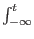)/Resistance .

Resistors are the bridge between normal voltage-mode devices and phase nodes. Some circuits may require introduction of resistors to get correct results. For example, assume a Josephson junction logic gate driving a CMOS comparator circuit. If the input MOS gate is connected directly to the junction, the DC operating point will be incorrect, as the comparator will see phase as input. However, if a resistor separates the MOS gate from the junction, the comparator input will be zero, as it should be.

There is (at present) a topological requirement that all phase nodes must be at ground potential. This means that for a network of Josephson junctions and inductors, there must be a ground connection to one of these devices. A nonzero voltage source connected to an inductor, which is connected to a resistor to ground, although a perfectly valid circuit, will fail. One must use the equivalent consisting of the voltage source connected to a resistor, connected to the inductor which is grounded. This satisfies the two topological requirements:

Rule 1
There must be a resistor between a voltage-mode device and a phase-mode device, no direct connections allowed.

Rule 2
Every phase-node subnet must have a connection to ground, so all phase nodes are at ground potential.

With this bit of information, and the warning that controlled sources can cause unexpected behavior, the DC analysis using thes technique can apply to general circuits containing Josephson junctions.

Of course, for this to work, no Josephson junction can be biased above its critical current or nonconvergence results. Both DC operating point and DC sweep are available, as is AC analysis. Noise analysis is available with the internal Josephson junction model. This new hybrid technique appears to be an important advance, which should avoid the long-standing need to use uic'' and ramp sources up from zero, and makes available DC sweep analysis, and for the first time in any simulator AC small-signal analysis.

See the Josephson junction model description for more information.Next: Josephson Junction Model Up: Superconductor Devices Previous: Superconductor Devices   Contents   Index
Stephen R. Whiteley 2019-03-16